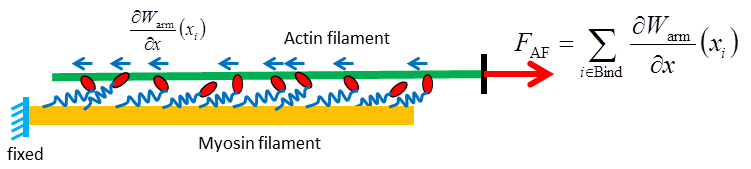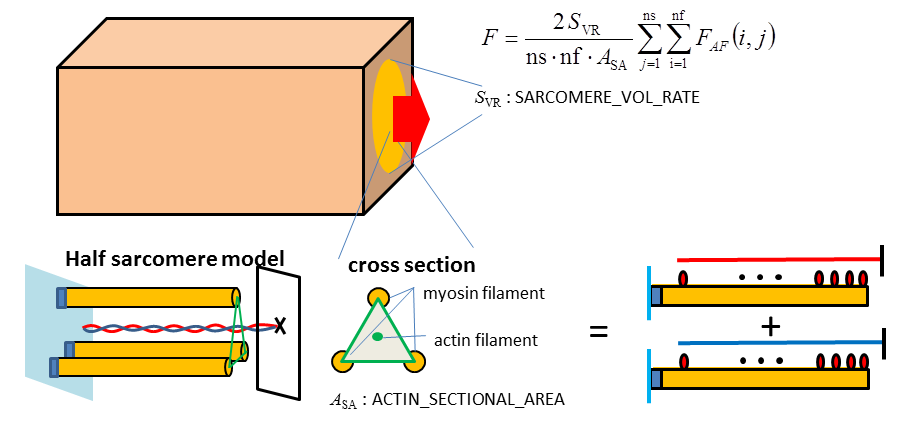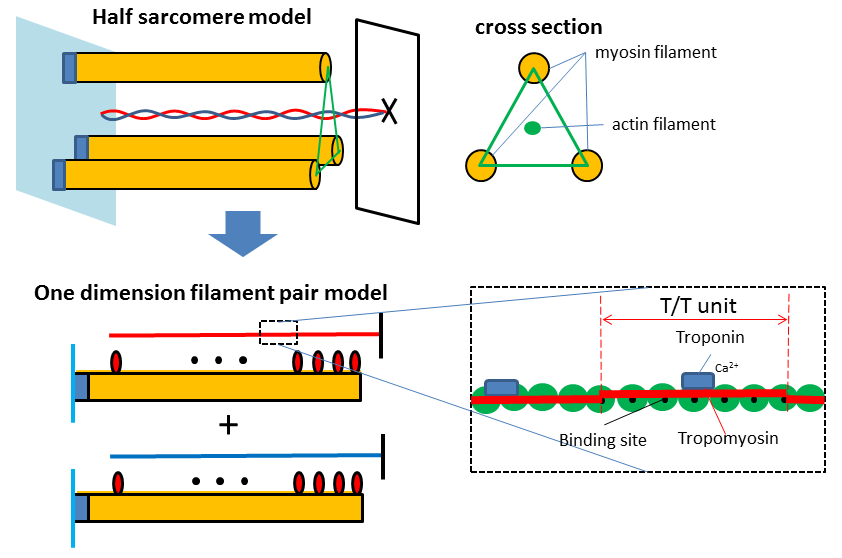## Definition of contractile force

### Arm stretch in a duration of binding states

XB Modeler calculates the myosin arm stretch x by taking the initial stretch, the events of head rotations and the shortening velocity du/dt into account. In the figure below, we assume that the myosin transfers from a no binding state to a binding state at time tbind. xstroke is the sum of contributions of the head rotations which occur after the time tbind. In XB Modeler, the function arm_stretch() retruns the arm_strech in nm for each myosin. And the functions spring_force(x) and spring_energy(x) return the force in pN and the strain energy in pN·nm, respectively, for a given arm stretch x in nm.### Contractile force for a filament pair model

In XB Modeler, we assume that the mechanical equilibrium between the force FAF acting at the actin free end and the net force of myosin arm springs is always attained.### Contractile force in the continuum

In the continuum model, the force F is given as the net force of the actin filament forces FAF on the unit sectional area perpendicular to the filament direction. XB Modeler assumes that the occupancy of sarcomere in the total sectional area is given by the parameter SARCOMERE_VOL_RATE, and that the triangular area per an actin filament is given by the parameter ACTIN_SECTIONAL_AREA. You can adjust these parameters through Edit Parameters in the menu bar and clicking on the Sarcomere tab. The number of filament pairs simulated in XB Modeler is given by the product of the number of samples (ns: # of samples) and the number of filaments (nf: # of filaments) in one sample. You can adjust these numbers in Simulation Setup dialog when you run a simulation. In the current version, there are no interactions between different filament pairs. However, the interaction will be implemented in the future version. This is why there are two indices to identify a filament pair.### Correspondence of an actin filament and the filament pair model in XB Modeler

In the real three dimensional arrangement, an actin filament interacts with three myosin filaments surrounding it. An actin filament is composed of two sequences of actin monomers, and the tropomyosin molecules wrap around binding sites with the myosin molecules along each sequence. The arrangement of the tropomyosin is controlled by the troponin that reacts with calcium ions and changes the configuration of tropomyosin to expose the biding sites. In XB Modeler, we assume that each triangle corresponding to an double helix actin filament that is composed of two filament pair models. That is why the factor two is multiplied in the numerator of the definition for “F”. You can specify the number of myosin molecules (NU_MF) along the myosin filament and the number of T/T units (NU_AF) along actin filament of XB Modeler through Edit Parameters in the menu bar and clicking the Sarcomere tab. According to the standard data from some literatures, the default values are set as NU_MF = 38 and NU_AF=32.